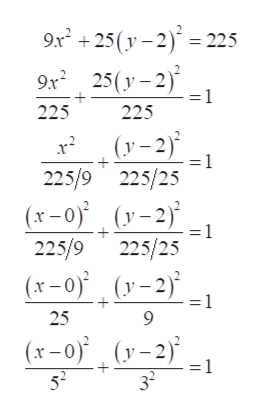# For Exercise, identify each equation as representing a circle, an ellipse, a hyperbola, or a parabola.• If the equation represents a circle, identify the center and radius.• If the equation represents an ellipse, identify the center, vertices, endpoints of the minor axis, foci, and eccentricity.• If the equation represents a hyperbola, identify the center, vertices, foci, equations of the asymptotes, and eccentricity.• If the equation represents a parabola, identify the vertex, focus, equation of the directrix, and equation of the axis of symmetry.9x2 + 25(y − 2)2 = 225

Question
11 views

For Exercise, identify each equation as representing a circle, an ellipse, a hyperbola, or a parabola.

• If the equation represents a circle, identify the center and radius.

• If the equation represents an ellipse, identify the center, vertices, endpoints of the minor axis, foci, and eccentricity.

• If the equation represents a hyperbola, identify the center, vertices, foci, equations of the asymptotes, and eccentricity.

• If the equation represents a parabola, identify the vertex, focus, equation of the directrix, and equation of the axis of symmetry.

9x2 + 25(y − 2)2 = 225

check_circle

Step 1

Consider the provided equation,

Step 2

Simplify the above equation and step of calculation is shown below,help_outlineImage Transcriptionclose9x +25(y – 2)' = 225 25(y – 2) = 1 9x 225 225 (y –2) = 1 225/9 225/25 (x-0) (y-2) 225/9 225/25 (x–0) (v-2)* 25 (r–0) (y-2) 52 = 1 32 fullscreen
Step 3

The simplified form of the equation represe...

### Want to see the full answer?

See Solution

#### Want to see this answer and more?

Solutions are written by subject experts who are available 24/7. Questions are typically answered within 1 hour.*

See Solution
*Response times may vary by subject and question.
Tagged in

### Other